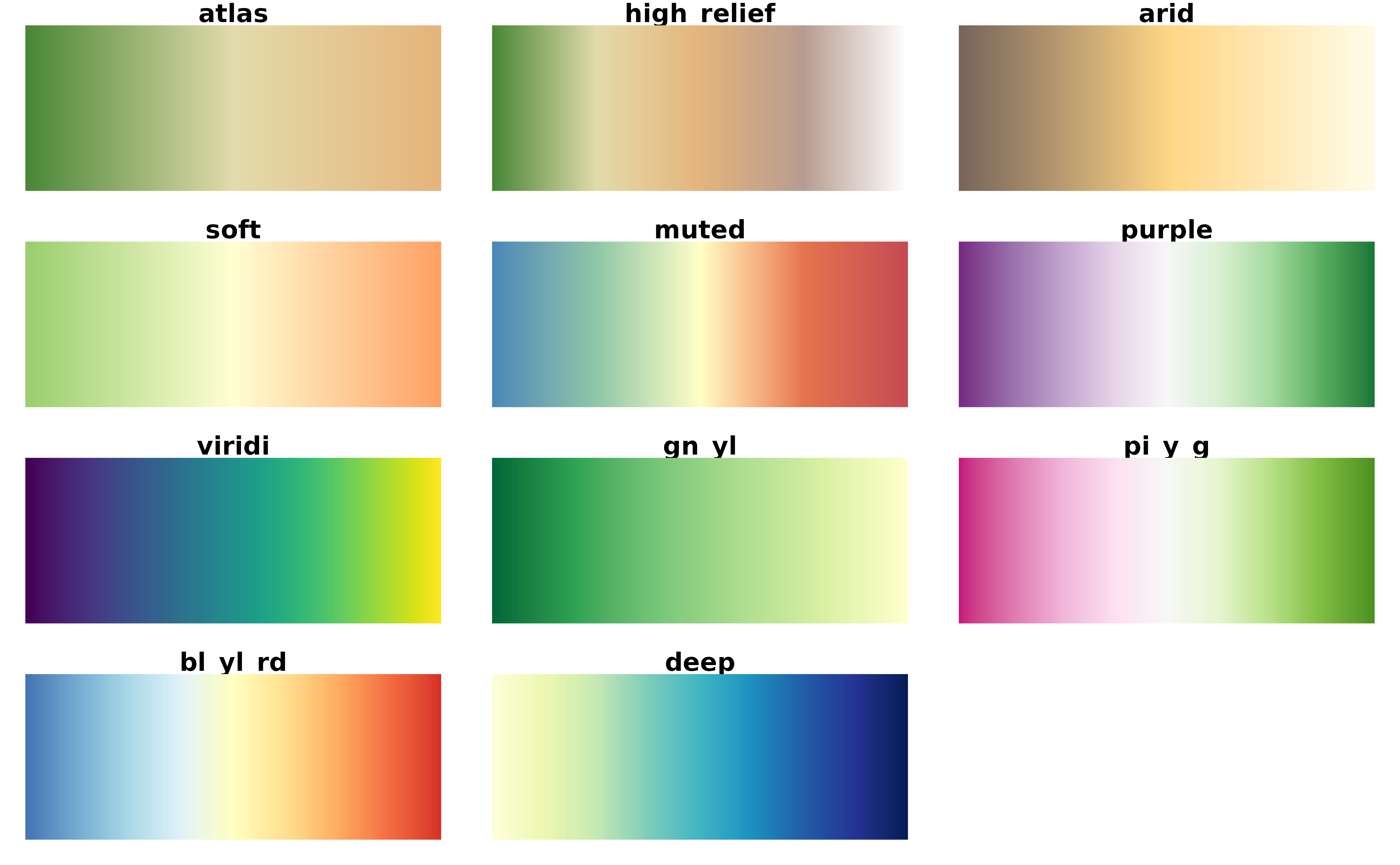Implementation of the gradient palettes provided by WhiteboxTools. Three scales are provided:

• scale_*_whitebox_d(): For discrete values.

• scale_*_whitebox_c(): For continuous values.

• scale_*_whitebox_b(): For binning continuous values.

Additionally, a color palette whitebox.colors() is provided. See also grDevices::terrain.colors() for details.

Additional parameters ... would be passed on to:

• Discrete values: ggplot2::discrete_scale()

• Continuous values: ggplot2::continuous_scale()

• Binned continuous values: ggplot2::binned_scale().

Note that tidyterra just documents a selection of these additional parameters, check the previous links to see the full range of parameters accepted by these scales.

## Usage

scale_fill_whitebox_d(
palette = "high_relief",
...,
alpha = 1,
direction = 1,
na.translate = FALSE,
drop = TRUE
)

scale_colour_whitebox_d(
palette = "high_relief",
...,
alpha = 1,
direction = 1,
na.translate = FALSE,
drop = TRUE
)

scale_fill_whitebox_c(
palette = "high_relief",
...,
alpha = 1,
direction = 1,
na.value = NA,
guide = "colourbar"
)

scale_colour_whitebox_c(
palette = "high_relief",
...,
alpha = 1,
direction = 1,
na.value = NA,
guide = "colourbar"
)

scale_fill_whitebox_b(
palette = "high_relief",
...,
alpha = 1,
direction = 1,
na.value = NA,
guide = "coloursteps"
)

scale_colour_whitebox_b(
palette = "high_relief",
...,
alpha = 1,
direction = 1,
na.value = NA,
guide = "coloursteps"
)

whitebox.colors(n, palette = "high_relief", alpha = 1, rev = FALSE)

## Arguments

palette

A valid palette name. The name is matched to the list of available palettes, ignoring upper vs. lower case. Values available are: "atlas", "high_relief", "arid", "soft", "muted", "purple", "viridi", "gn_yl", "pi_y_g", "bl_yl_rd", "deep".

...

Arguments passed on to ggplot2::discrete_scale, ggplot2::continuous_scale, ggplot2::binned_scale

breaks

One of:

• NULL for no breaks

• waiver() for the default breaks (the scale limits)

• A character vector of breaks

• A function that takes the limits as input and returns breaks as output. Also accepts rlang lambda function notation.

labels

One of:

• NULL for no labels

• waiver() for the default labels computed by the transformation object

• A character vector giving labels (must be same length as breaks)

• An expression vector (must be the same length as breaks). See ?plotmath for details.

• A function that takes the breaks as input and returns labels as output. Also accepts rlang lambda function notation.

limits

One of:

• NULL to use the default scale values

• A character vector that defines possible values of the scale and their order

• A function that accepts the existing (automatic) values and returns new ones. Also accepts rlang lambda function notation.

expand

For position scales, a vector of range expansion constants used to add some padding around the data to ensure that they are placed some distance away from the axes. Use the convenience function expansion() to generate the values for the expand argument. The defaults are to expand the scale by 5% on each side for continuous variables, and by 0.6 units on each side for discrete variables.

minor_breaks

One of:

• NULL for no minor breaks

• waiver() for the default breaks (one minor break between each major break)

• A numeric vector of positions

• A function that given the limits returns a vector of minor breaks. Also accepts rlang lambda function notation.

n.breaks

An integer guiding the number of major breaks. The algorithm may choose a slightly different number to ensure nice break labels. Will only have an effect if breaks = waiver(). Use NULL to use the default number of breaks given by the transformation.

nice.breaks

Logical. Should breaks be attempted placed at nice values instead of exactly evenly spaced between the limits. If TRUE (default) the scale will ask the transformation object to create breaks, and this may result in a different number of breaks than requested. Ignored if breaks are given explicitly.

alpha

The alpha transparency, a number in [0,1], see argument alpha in hsv.

direction

Sets the order of colors in the scale. If 1, the default, colors are ordered from darkest to lightest. If -1, the order of colors is reversed.

na.translate

Should NA values be removed from the legend? Default is TRUE.

drop

Should unused factor levels be omitted from the scale? The default (TRUE) removes unused factors.

na.value

Missing values will be replaced with this value.

guide

A function used to create a guide or its name. See guides() for more information.

n

the number of colors ($$\ge 1$$) to be in the palette.

rev

logical indicating whether the ordering of the colors should be reversed.

## Value

The corresponding ggplot2 layer with the values applied to the fill/colour aesthetics.

terra::plot(), ggplot2::scale_fill_viridis_c()

See also ggplot2 docs on additional ... parameters:

• scale_*_terrain_d(): For discrete values.

• scale_*_terrain_c(): For continuous values.

• scale_*_terrain_b(): For binning continuous values.

Other gradient scales and palettes for hypsometry: scale_color_coltab(), scale_cross_blended, scale_hypso, scale_terrain

## Examples

# \donttest{
filepath <- system.file("extdata/volcano2.tif", package = "tidyterra")

library(terra)
volcano2_rast <- rast(filepath)

# Palette
plot(volcano2_rast, col = whitebox.colors(100))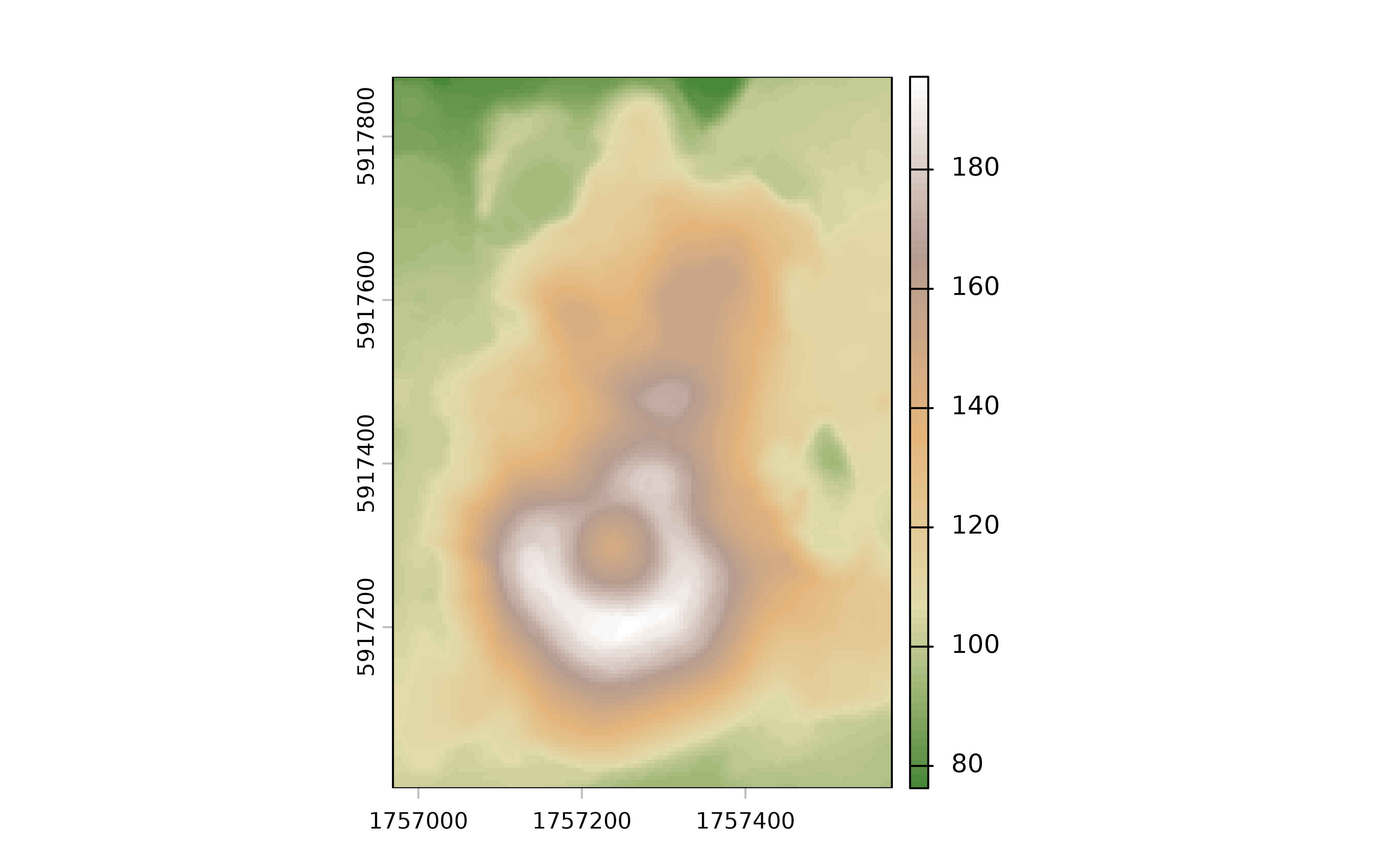library(ggplot2)
ggplot() +
geom_spatraster(data = volcano2_rast) +
scale_fill_whitebox_c()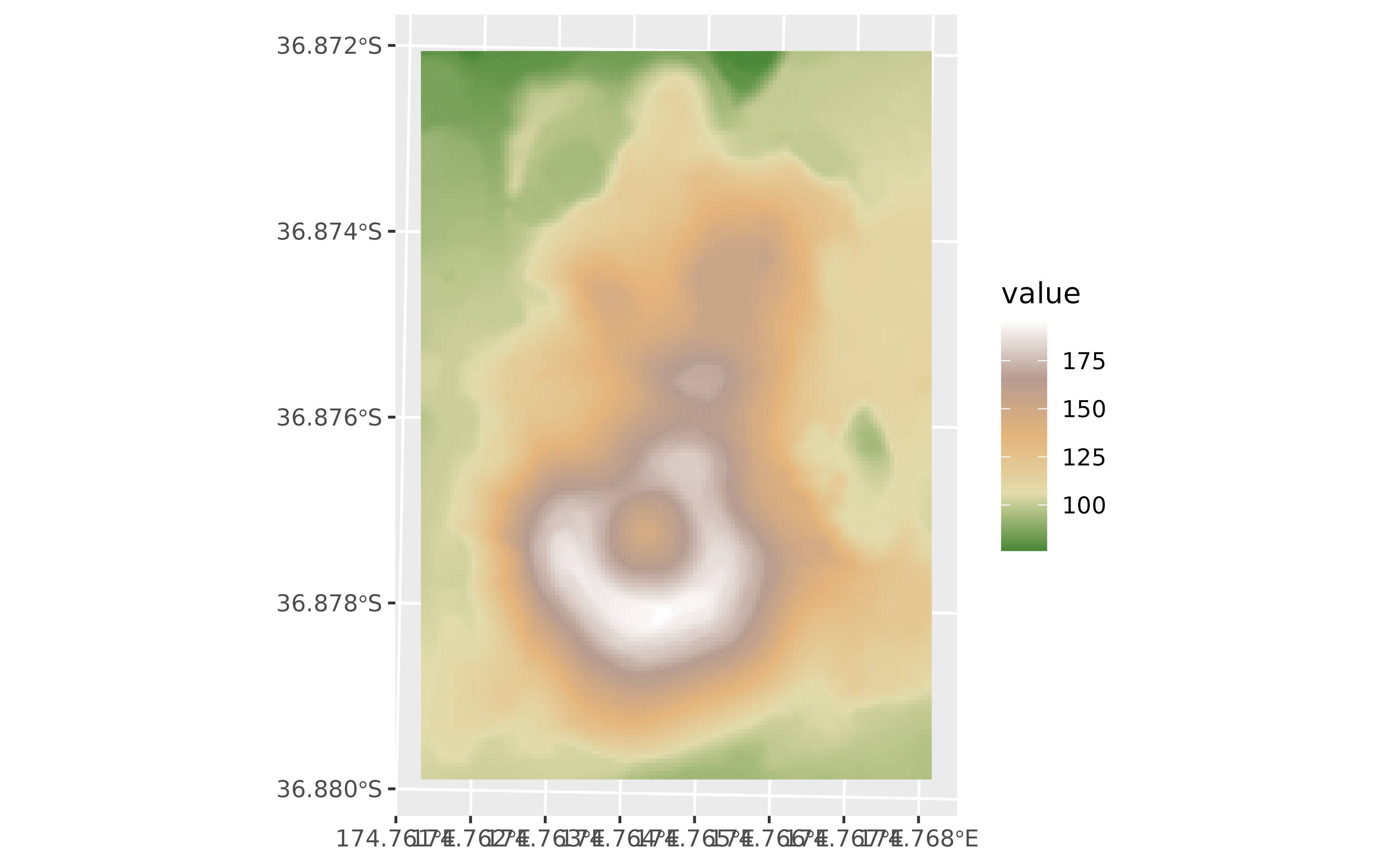# Binned
ggplot() +
geom_spatraster(data = volcano2_rast) +
scale_fill_whitebox_b(breaks = seq(70, 200, 10), palette = "atlas")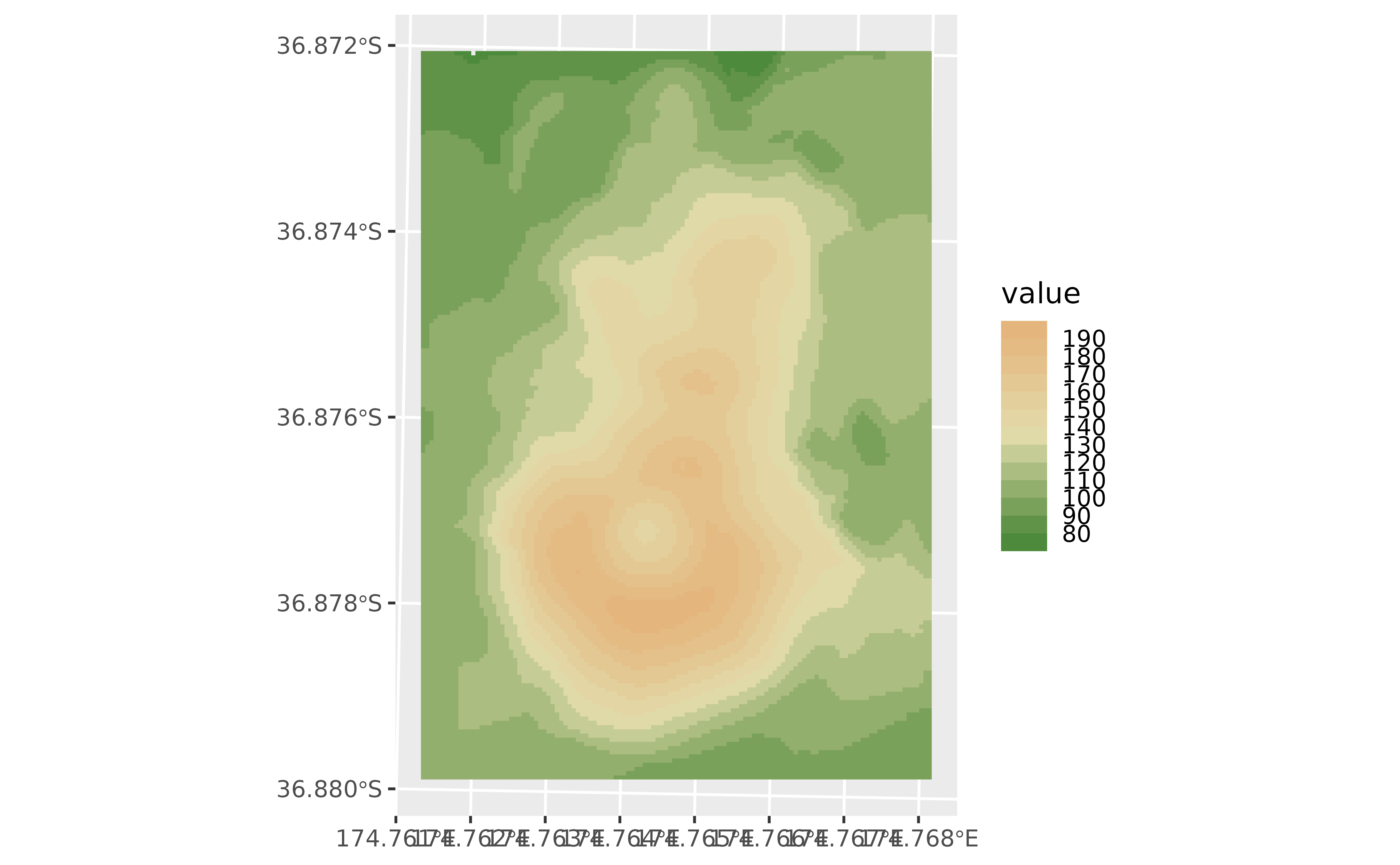# With discrete values
factor <- volcano2_rast %>% mutate(cats = cut(elevation,
breaks = c(100, 120, 130, 150, 170, 200),
labels = c(
"Very Low", "Low", "Average", "High",
"Very High"
)
))

ggplot() +
geom_spatraster(data = factor, aes(fill = cats)) +
scale_fill_whitebox_d(na.value = "gray10", palette = "soft")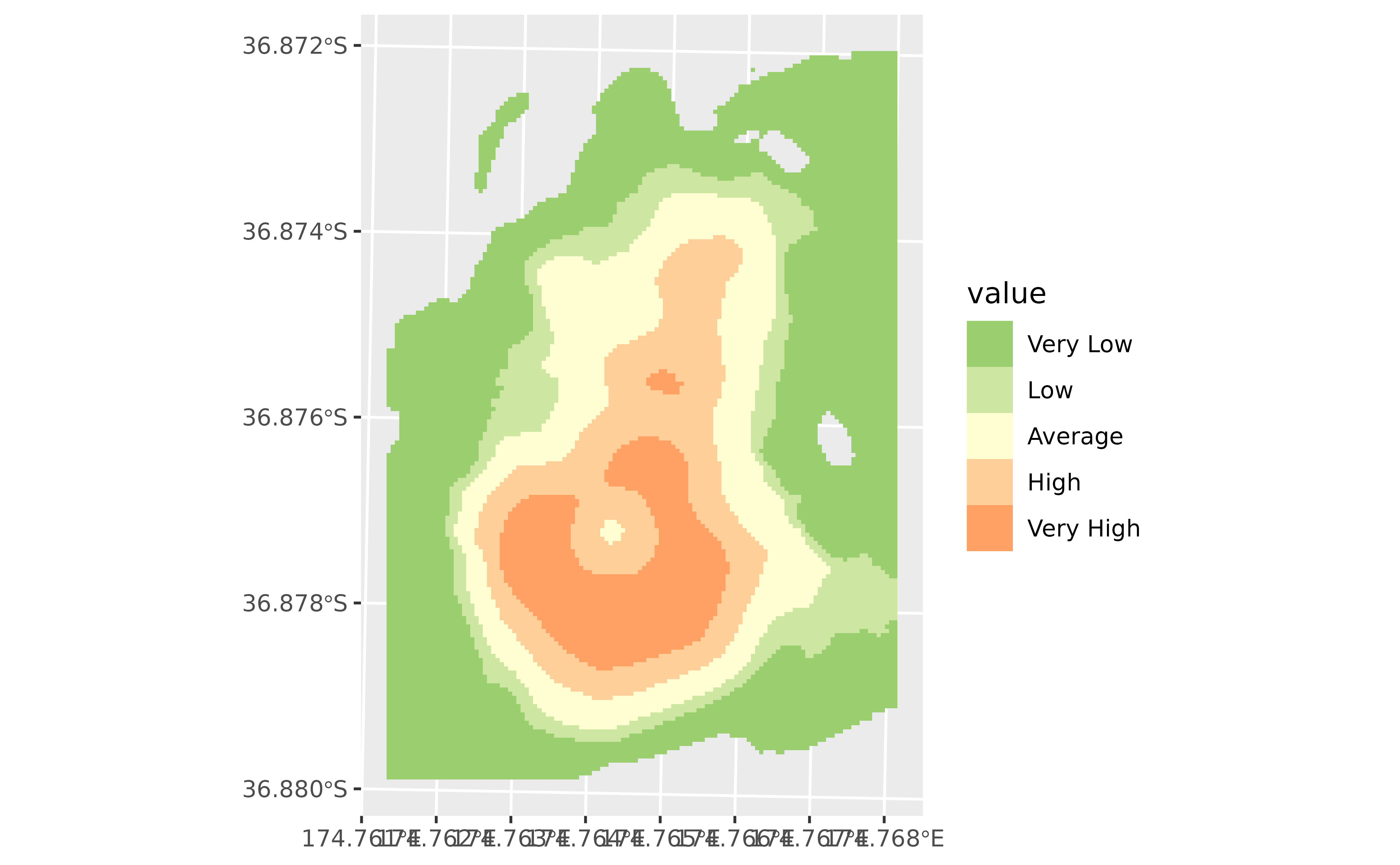# }

# Display all the whitebox palettes

pals <- c(
"atlas", "high_relief", "arid", "soft", "muted", "purple",
"viridi", "gn_yl", "pi_y_g", "bl_yl_rd", "deep"
)

# Helper fun for plotting

ncols <- 128
rowcol <- grDevices::n2mfrow(length(pals))